International Journal of Ophthalmology & Eye Science (IJOES)   Eye and Light Science    IJOES-2332-290X-S9-002

### Lens Fluid Displacement and Accommodation

Garner WH1*, Burns WR2

1 Bioptics Research, Eastport ME, USA.
2 Encore Vision, Inc., Fort Worth, TX, USA.

*Corresponding Author

William H. Garner,
Bioptics Research, Eastport ME, USA
E-mail: dr_garner@bioptics-research.com

Received: July 22, 2016; Accepted: November 09, 2016; Published: November 15, 2016

Citation: Garner WH, Burns WR (2016) Lens Fluid Displacement and Accommodation. Int J Opthalmol Eye Res, S9:002, 9-20. doi: dx.doi.org/10.19070/2332-290X-SI09002

Copyright: Garner WH© 2016. This is an open-access article distributed under the terms of the Creative Commons Attribution License, which permits unrestricted use, distribution and reproduction in any medium, provided the original author and source are credited.

Abstract

In this study, the biomechanics of the human lens geometry were numerically analyzed to quantitate micro-fluidic movement of lens fiber cells. Literature values were collected to develop a lens geometry paradigm related to optical output for both distant and near vision. We show that our numerical methods can successfully quantitate the micro-fluidic flow of lens fiber cells. Small changes in the lens fluidic movement responsible for optics are confined to a cortical volume element that appears compromised by lens modulus changes. The result imply that aging of the lens is accompanied by an almost constant inward equatorial movement at 21.2 ± 0.002 microns/∂D. This creates a corresponding fluid volume of 1.74 ± 0.17 μL/∂D that is displaced per diopter of accommodation. A possible presbyopia lens treatment that achieves an acceptable accommodative end-point requires an estimate of the active pharmacological dose level. A therapeutic delivery requirement involving the entire lens mass may therefore be substituted by a treatment that targets the outer cortical region (or <2% of the total lens protein).

1.Keywords
2.Introduction
3.Materials and Methods
3.1.Estimated Lens Elliptical Geometric Values
3.2.Distance Vision Elliptical Geometric Best-Fit Values: aD and baD
3.3.Calculation of the Lens Microfluidic Optical Limit Values: VoN, VFComp, and VFC
4.Results
4.1.Lens Fluid Volume Displacement During Accommodation: ∂VoNfit
4.2.Calculation of the Lens Fiber Cell - Laminar - Fluid Layer Volume (Vlam) and its Possible Effects on Loss of Accommodation
4.3.Calculation of Possible Lens Drug Level Requirements to Restore Accommodation
5.Discussion
5.1.Encore Vision completes a Phase I/II Trial for Presbyopia in the USA (NCT02516306)
6.Conclusions
7.Acknowledgments
8.References

Keywords

Biomechanics; Lens; Presbyopia; Vision; Refraction; Optics; Modulus; Ocular; Hagen-Poiseuille; Fahraeus-Lindqvist Effect.

Introduction

The human lens has an integrated cell structure. The anatomy of the lens includes long lens fiber cells that may act as micro-fluidic channels [1, 2]. These fiber cells wrap around the outer perimeter region of the lens during cell differentiation and elongation . An estimated 250,000 fiber cells initially change in length with the accommodation. The anatomy and structure of the lens fiber cells have been described in the literature [2, 4].

The human lens cell structure determines functional optical output needed for accommodative amplitude. As shown in Figure 1, ∂D (solid line), the condition referred to as presbyopia entails a progressive degenerative loss of near vision [5, 6]. Almost 30% of the world’s present population is over 45 years of age and may experience presbyopia symptoms. A current question involves the possibility of reversing the loss of accommodative amplitude, so as to offset the need for reading glasses. The other possibility is that treatment may be limited to inhibition of progression (e.g., intervention at 40 years). The discovery of a successful presbyopia treatment has many requirements, which are beyond the scope of this paper. Drug identification (i.e., a rational approach) requires a target (i.e., the lens) and some hypothesis regarding the nature of the pathophysiological defects (i.e., underlying presbyopia). Helmholtz originally suggested increasing lens stiffness .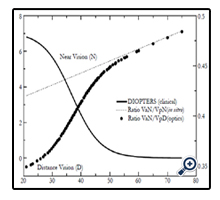Figure 1. Graphic plot of age-related changes (years) in diopter-accommodative amplitude, indicated with a solid line (∂D: diopters-left axis), and the effects of lens growth (dashed line) on the in vitro measured VaN/VpN volume axial ratios (arbitrary units, rightaxis). The corresponding VaD/VpD ratios are indicated by the solid black circles.

Lens proteins are retained throughout a person’s lifetime ; therefore, they can undergo accumulative posttranslational changes, such as oxidation of the protein and small molecules [9-11]. Oxidation produces protein disulfide cross links (PSSP) that are probably involved in the measured increase of the lens modulus (i.e., cytosol matrix stiffness) [12, 13]. The current hypothesis is that the nuclear portion of the lens is mainly responsible for presbyopia [14-16]. The nucleus shows a massive increase in the modulus of the human lens with aging , which fits with Helmholtz’s theory. The nuclear volume, like the entire lens, increases with aging.

The anatomical diagram developed by Kuszak et al.,  of the microfluidic channels created by the long lens fiber cells represents a key insight motivating this work. Given their described anatomical structure, changes in the lens geometry would be accompanied by a corresponding displacement of the lens fiber cell cytosol. This suggests an alternative hypothesis: that displacement of cytosol fluid of the cortical fiber cells is responsible for the geometry change of the lens to increase its optical power .

To the best of our knowledge, no attempt has been made to use microfluidics to understand accommodation [19-23]. Unfortunately, no a priori method exists for quantitating lens geometric axis parameter values, other than using empirical measurements. The data analyzed here are obtained from clinical patients  and biochemical studies  with different units of measure (diopters or μL, respectively). As developed here, the key link is the thick lens optical formula (TLF), where input lens geometry (i.e., structure) determines the accommodative output (i.e., function).

Materials and Methods

Numerical lens methods were used to approximate the accommodative amplitude dynamics to improve our understanding of the pharmacology for a possible presbyopia treatment. The mathematical terms used in the numerical methods are shown in Table 1.Table 1. Definition of Terms.

In a general view, the mathematical approach we derived mimics the mechanical compression (an external force, e.g., zonular biomechanical system) of a sphere (elastic) that is acted upon between two parallel plates, resulting in an ellipsoid (with an increasing surface area) [25, 26]. Removal of the applied external force causes the spherical shape to then reform to minimize internal forces (i.e., a lower surface area). This effect is much like the forces acting on the lens for accommodation (i.e., Helmholtz), but rather than a stress force (i.e., plates on the sphere), the human optical system applies shear (i.e., zonular attachment) forces that act at the equatorial vertex. The lens is connected to the ciliary muscle by a series of zonular fibers that originate at the ora serrata . Removal of the lens from the eye causes it to assume the accommodated geometry. The in vitro measurements  give us the lens volume, diameter, and lens thickness changes during aging. We must then calculate the lens dimensions under those zonular shear forces that represent the dis-accommodated lens state, which is much like stretching the lens in a mechanical device [28, 29].

An example of term generation is obtained by considering the human lens volumetric structure as nearly an oblate ellipsoid  with a major axis (“a”) and a minor axis (“b”), separated into anterior and posterior regions (indicated in Figure 2). However, a simple qualitative examination of the lens anatomical profile, as shown in Figure 2, suggests that the aspect ratio of the anterior volume (VaD) to the posterior volume (VpD) has a value of less than one. The distance (“D”) vision ellipsoid (or lens) posterior (“p”) volume (“V”), termed “VpD”, is calculated with a majoraxis radial distance value (“aD”) and a minor posterior axis distance value (“bpD”) distance value. The in vitro data provides us with the near vision geometric values: major axis, aN (with “N” for near), and similarly, the minor “b” axes: anterior baN and posterior terms bpN, respectively. We used the best-fit functions without modification, as described in previous studies [8, 24, 30]. Each of the cited references refers to functions derived from large data sets (N>100).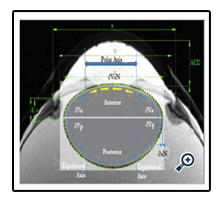Figure 2. A) Ultra-high-field 7.1 T MRI of a 40-year-old human donor eye with in-plane resolution of 80x80 μm) . The published MRI was labeled to indicate regions of interest within the described text. The equatorial axis acts as the fluid storage volume at the anterior volume (∂VaD ) and posterior volume elements (∂VpD ) under zonular tension (distance vision, D). The (visual) central polar axis accumulates the designated fluid displacement volume, VoN, when zonular tension is released (near vision, N). The blue and yellow arrows indicate mass fluid flow to VoN. Use of the lens geometric values aDfit, baDfit, and bpDinvitro , as described in the text, provides a qualitative lens profile alignment, highlighted by the green dashed line. This figure also demonstrates the subtle 60-micron inward equatorial movement (∂xNfit described in the text) to displace 2.5 μL fluid (VoN) for a +2∂D improvement in accommodation.

We know that most of the accommodation takes place at the anterior surface [31, 32]. Fisher reported that the aspect ratio is nearly constant with age while dis-accommodated (i.e., a lower lens power state for distance vision); Equation (1) . The Fisher observation conveniently allows us an initial estimate of VpDest, with Equation (2) or with Equation (3).The in vitro data (dashed line shown in Figure 1) we used also included measurement of the VaN to VpN ratio as the lens ages and shows similar ratios to those noted by Fisher. The corresponding lens anterior VaDest value can then be estimated using Equation (4), where anterior and posterior volumes are linked with Equation (5) . Likewise, each geometry term is interrelated to the others, as shown by Equations 6–9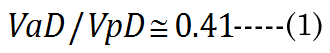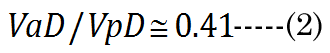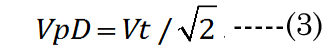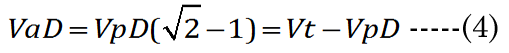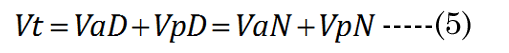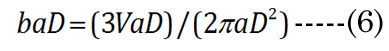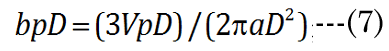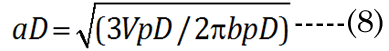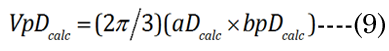EQN (23) certainly allows an estimate of the lens posterior volume value,VpDest (and thus VaDest), but it does not give us the individual major and minor axis values for aD, baD, and bpD. A functional analysis that considers the optics can instead provide these determinant values. For this purpose, we developed a unique parametric equation for examination of lens parameter geometry.

Estimated Lens Elliptical Geometric Values

The change in the geometry of the anatomical minor anterior axis generates a lens thickness change (boN) between the accommodated and dis-accommodated optical states. We examined how the geometric shape affects accommodation by developing a simple parametric Equation (10) to satisfy Equation (11) for small changes in sphere radius (Rs). A lens spherical volume (Vt = 150 μL; Rs = 3.3 mm) was transformed according to f(∂xi), using EQN (10), into an equivalent (ellipsoidal) dimension, as shown in Figure 3. The major axis (aDest), using f(xDest), provides an age-dependent estimation of the maximal (distance vision) equatorial posterior volume (VpDest) at f(xDest) with an iteration of ∂xi, according to Equation (12), where aDest=Rs+xDest. As shown in Figure 3 (dashed line), the ultimate purpose of the VaD/VpD ratio (note that the y-axis value is 0.414 when ∂x = 1.26 mm) is to maximize the internal fluid into the equatorial cortical lens posterior region (VpD) when an external (zonular) force is applied. In our lens optical model, the stretching force is not located at the spheroidal equator. The posterior minor axis bpDest was calculated with ay(∂xi) value substitution, using Equation (13). The function of ∂xi, with EQN 10: f(∂xi), was resolved as approximately Rs/2 or specifically (f(∂xi)) =Rs/1.87 for aDest.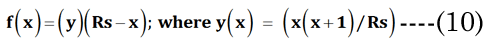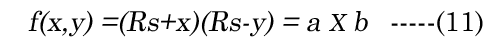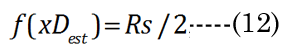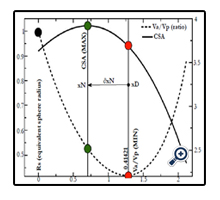Figure 3. Numerical method for estimation of major aDest and aNest lens axes. This example is constructed for a hypothetical (low modulus) lens. An equation term was derived (see EQN(10): f(∂xi)) to transform a spheroid to an ellipsoid of equal volume. Equations EQN (12) and EQN (13) supply our variables aDest and bpDest, respectively. The Vp was estimated using EQN (9), with Va determined by the difference from EQN (4).The ellipsoid radius (Rs + ∂xi) was calculated with a numerical spheroid (Rs, black closed circle) “stretching” over a range (∂xi) 0 to 2 mm. The dashed line describes the calculated Va/Vp ratios. The minimum Va/Vp value is the resultant calculated VpDest optimal value (solid red circle).We see that the optimal posterior volume (Vp) is maximized (left-axis value 0.414) at EQN(10): f(∂xi) approximately Rs/2. This “stretch” point is the most efficient point for distance vision (xDest). We also see that the lens cross sectional area (CSA) (mm2 right-axis) is not maximal at xDest. Near vision requires a maximal CSA for refractive power. Using the same values from EQN(10): f(∂xi), we constructed the change of CSA with ∂xi indicated by the solid line. The optimal point (green solid circle) marks the point of maximal CSA for lens accommodation (xNest). The calculation also shows the optimal range of equatorial movement (∂xN) for best lens performance (552 μm).

The final determination of the ∂xi for the aN value depends on the value of f(∂xi), within optical boundary limits: (xNest, near vision) or (xDest, distance vision), again as graphically shown in Figure 3. The actual lens optical performance (i.e., accommodation) stems from the change in the anterior minor axis values, baD and baN, which link aD to the equatorial inward movement (∂xi) of aN, to maximize the lens cross-sectional area (CSA) for light refraction. Applying f(xi) with Equation (14), where bpN ≌ bpD in Equation (15), we generate the solid black line shown in Figure 3. The lens CSA results with f(∂xi) are shown in Figure 3, with ∂xi values ranging from 0 to 2.5 mm. We observe that the maximal CSA near-vision optics occur at ∂xi = 0.713 mm, when f(xi) = Rs/3.45 mm. Disregarding lens modulus effects, the estimated range of the lens equatorial inward movement is 552 μm (microns).

Obtaining an implicit maximal optical effect (i.e., fluid displacement to the optical axis) would require that the posterior minor axis, bpD, does not significantly change between near or distance vision (other than due to growth); therefore, we assume that bpD ≈ bpN . At least, this is our (teleological) argument to simplify the numerical analysis, as far as defining the nature of cause and effect regarding the validity of the paradigm assumption. The paradox remains that, despite the constant of the posterior ellipsoid dimension, it (i.e., the posterior region) is the main source of displacement fluid (later termed VoN) needed for accommodation. This subtle biomechanical process maximizes the possible fluid mass movement to the polar axis to affect accommodation. As such, the posterior volume is very important to lens-adaptive optics.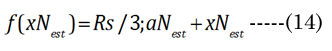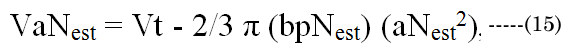Distance Vision Elliptical Geometric Best-Fit Values: aD and baD

The major axis aNinvitro value was determined  using Equation (16). Minor axes baNinvitro and bpNinvitro values were calculated using the corresponding lens aspect ratios (VaN/VpN); first using Equation (17): The in vitro VaN/VpN ratio values determined are shown in Figure 1 (dashed line). Second, EQN (17) was used with the total lens volume (Vt) calculated with Equation (18) VaN is determined with Equation (19). These calculated values were then used to determine baN|VaN, aN and bpN|VpN, aN, similar to equations EQN(6) and EQN(7), respectively.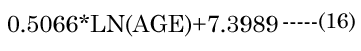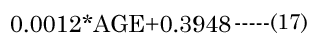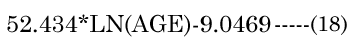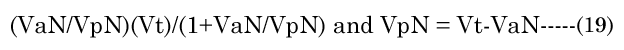The optical defined inward motion (∂xN) affected by the lens modulus was determined using the standard thick lens formula (TLF) derived by Morgan, Equation (20). The terms fD and fN are the distance and near vision focal lengths, respectively . We first determined fN from the in vitro results. R1N, R²N, and tN were correspondingly calculated using the previously introduced terms (aNinvitro and bpNinvitro) with Equation (21)& Equation (22). However, the fD term requires an iteration of ∂xi (inward equatorial axis movement) to find a best fit value that corresponds (error limit < 0.0001 mm) to the clinically determined amplitude (∂D) , according to Equation(23) . The results in Figure 1 show that the ∂D change demonstrates a progressive loss of accommodation with increasing age that corresponds well with other published literature values . We assumed that the aqueous refractive index (naq= 1.33) and lens cortical region refractive index (nlens = 1.418) are nearly homogeneous . In reality, the lens has a subtle anisotropic gradient refractive index (GRIN), but the precise refractive structure is still unknown, although it is probably similar to that shown by Teichman et al., . The overlooking of GRIN should not be considered a major limitation in the final tabulated values that we present, as the GRIN effects would only slightly reduce our final calculated lens movement (∂xN) values [31, 37-39].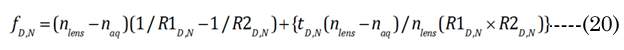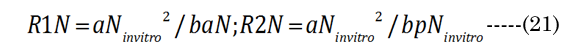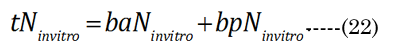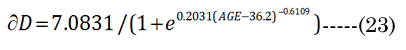The numerical fitting method used a non-linear, generalized, reduced-gradient numerical method, via Equation (24). Intermediate values were calculated for R1i, R²i, and ti during the fitting procedure. The increase in lens thickness, ∂boNfit, was determined using Equation (25), with term substitution in EQN (6). The aDfit value at each age was determined with initial estimates of ∂xi, with final convergence to determine the best-fit values to ∂D(age), according to Equation(26)(shown in Figure 4 by the green closed circles). The calculated values are compared in Figure 4 to the corresponding age-matched aNinvitro values. The final numerically derived aDfit values reflect the modulus- sensitive (R1D, R²D) curvature and lens thickness (tD) parameters for the determination of fD.

The dis-accommodated parameters at this point give a relatively good lens profile cross-section agreement with an MRI acquired from a 41-year-old patient (dashed green line in Figure 2). The parameter determination gives us the dimensions required to describe the 3-D volumetric profile of the lens for distance vision. The resting (i.e., with the applied zonular tension) optical state is set for distance vision (D) that is not lost with age (i.e., the lens paradox) .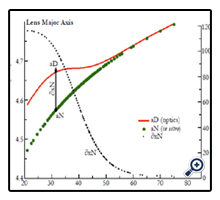Figure 4. Graphic showing the age-related loss of the lens major axis equatorial movement (mm left-axis) between distance aDfit (red line) and near vision aNinvitro(green line). The effects of increasing lens modulus are shown with the decreasing magnitude of ∂xN (vertical arrow) with age and indicated by small black circles with an expanded μm scale (rightaxis).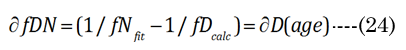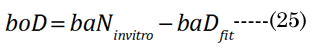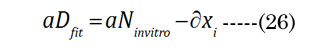Calculation of the Lens Microfluidic Optical Limit Values: VoN, VFComp, and VFC

The optical displacement volume (VoN) of the lens fluid upon accommodation (D to N) was calculated with Equation (27),using aN in vitro and boNvalues. The lens cross-sectional area increased with near vision, using Equation (28), where Equation (29) and Equation (30).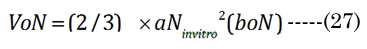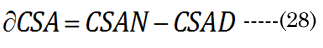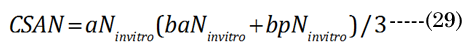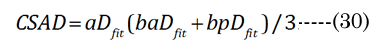A conceptual laminar flow term lambda (λ), derived from the Hagen-Poiseuille flow from the Navier-Stokes equations, was calculated using Equation (31) ; when CSAD equals CSAN, then λ is 0. We assumed, for our purposes, that the newly formed (cortical) lens fiber cell exhibited a Newtonian steady laminar flow through a long cylindrical pipe of a constant cross-section. The partial volume of the fiber cell laminar layer (Vlam) within VoN was determined by Equation (32). The equatorial lens fiber cell compression volume (VFComp >VoN) was calculated using Equation (33). The total (polar and equatorial) lens compression volume (VFC) was determined via Equation (34)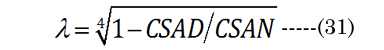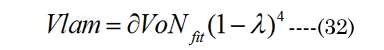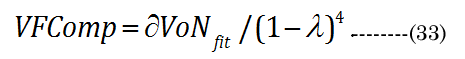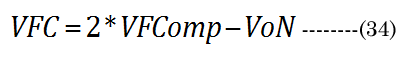Bernoulli’s principle declares that the energy loss is proportional to the change in hydrodynamic pressure (Pai). The idealized Hagen-Poiseuille unit volume is illustrated in Figure 5 for a classical pipe. The Hagen-Poiseuille Equation (35) Equation (35): expresses the flow rate (∂V/∂t) of a volume element (πR²/Δx), with ∂V being equivalent to our term VoN. The flow rate is sensitive to the fluid viscosity (η, resistance force) and the CSAD (πR²) of the applied inward pressure(Pai = Pao×CSAN/CSAD) over a pipe length (R²/Δx). The outward lens pressure (Pao) was inferred from the lens modulus)  (using our CSA values calculated above). The ratio Pao to ∂VoN increases exponentially (Pao/VoN = 0.124eage*0.20) with age. The necessary inward capsule pressure (Pai) acts on the lens for accommodation. Therefore, accommodation only occurs if Pai is greater than Pao. Resistance to the flow rate (VoN/∂t) is proportionally reduced with an extant lower-viscosity outer laminar layer (thickness λ> 0). The Hagen-Poiseuille unit volume, illustrated in Figure 6, can be calculated using our values determined for VoN, ∂xN, and aDfit, accordingly, with Equation (36)&Equation (37).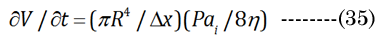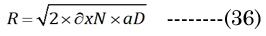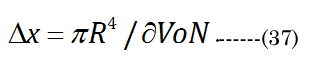In summary, i) aN, baN, and bpN were obtained from published in vitro measurements; ii) The inward equatorial movement ∂xNfit = aDfit-aNinvitro and baNinvitro was calculated (bpD = bpN) using the ∂D and the thick lens formula; iii) The change in the lens crosssectional area (∂CSA) was calculated from geometric measurements; iv) The fluid displacement volume (VoN) was calculated from the values of aNinvitro and lens thickness increase (boN); v) A factor λ was derived from CSAD and ∂CSA (CSAD-CSAN). vi) The laminar layer volume (Vlam) was subsequently calculated using Vlam = ∂VoN(1-λ)4 ; vii) The equatorial fiber cell compression volume (VFComp) was determined using VoN/(1-λ)4; and viii) fiber cell’s inner protein “core” volume (VFC) was determined using VFC = 2 (VFComp) - VoN.

The results are reported as the mean ± standard deviation (SD) (Microsoft Excel® for Mac version 14.6.4). Error estimates (SD) are determined from the value variation over the age range of 21–55 years. The calculated values are reported with three significant figures, since the changes are small and derived from the average of experimental studies (with respective N values shown in the table). All the derived numerical data (i.e., interpolation) demonstrate a smooth function that closely approximates the experimental results (data not shown). The GRG non-linear bestfit solutions were calculated (no constraints imposed) using the Solver tool in Excel version 15.27 (Microsoft, Redmond, WA).

Results

The calculated values aDfit, baDfit, and bpDfit provide the qualitative lens profile alignment shown in Figure 2 (MRI cross-section) as the dashed green line. The comparison appears to corroborate the numerical methods described for distance vision lens geometry.The resulting VaDfit to VpDfit ratio values are not constant with age as shown by the closed black circles in Figure 1; however, the "magic" ratio, 0.414, corresponds closely to the mid-point of accommodative amplitude loss (∂D at 40years).

Disregarding the increasing lens modulus our ad hoc EQN (10) predicted a larger inward radial movement (550 μm) than was defined from TLF using the clinical values (116 μm). The actual change in distance to near-focal length ∂fDN was calculated using the clinical change in accommodation (∂D). The final ∂xNfit values are marked as small black closed circles in the right axis of Figure 4, with dimensions of μm. Figure 4 (green closed circles) also shows the fitted values of aDfit, determined by clinical measurements (∂D). The aDfit value allows the calculation of baDfit shown in Figure 5. From 21 to 55 years, the boN to ∂xNfit ratio value is nearly a constant value of 1.67 ± 0.01 μL/mm (irrespective of modulus) and offers a 67% amplification of equatorial movement (∂xN) to the lens thickness change (boN), which is essential for increased ∂CSA and the resultant lens optical power.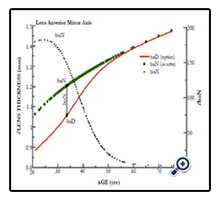Figure 5. Graphic showing the age-related loss of the lens minor axis anterior movement (mm, left axis) between distance baDfit (red line) and near vision baNinvitro (green line). The effect of increasing lens modulus diminishes the lens thickness (boN = tN-tD) magnitude, along with the accommodative amplitude. The metric boN is indicated by small black circles with an expanded μm scale (right axis).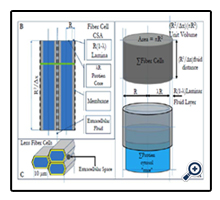Figure 6. A) The individual calculation of the laminar layer (Vlam , marked gray) and protein cytosol core protein (marked blue) components. B) The Hagen-Poiseuille unit volume can be rescaled to more closely match the anatomical lens fiber cell dimensions, at approximately 23 μm2 × 4500 μm (calculated) . The fiber cell CSA illustration denotes the respective compartments of the idealized Hagen-Poiseuille unit volume, with reference to the fiber cell membrane and its relationship to the extracellular compartment (passive fluid flux to the aqueous humor). C) The illustrated Hagen-Poiseuille unit volume “pipe” is actually composed of hexagonally shaped lens fiber cells that occupy a total sum (Σ) volume of VFComp. At 21years, around 250,000 cortical fiber cells are estimated to be responsible for VFComp.

As shown in Figure 5, a similar effect to aDfit occurs with baDfit with aging, to reduce lens thickness (boN indicated by solid red line) magnitude upon accommodation (The values of boN (closed black circles) with dimension of μm are shown in the right axis). As previously stated above, our numerical paradigm has no required change in the posterior minor axis. The distance-vision best-fit results shown in Figure 4 (aDfit) using clinical optics (∂D) indicate a gradually reduced inward radial movement ∂xN with age, but with a nearly constant 0.0213 ± 0.002 mm/∂D overall. Use of the best-fit value ∂xN determined at approximately 40 years reveals that the magnitude of inward movement (∂xN) is less than 60 microns for +2.4 ∂D accommodation, as shown in Figure 2. The corresponding boN-associated increase in lens thickness was only 82 μm.

Lens Fluid Volume Displacement During Accommodation: ∂VoNfit

Importantly, the numerical methods we have developed allow the transformation of previous literature data sources; the magnitude of VoN decreases with aging from 21 to 53 years, from 8.1 to 0.4 μL, respectively, with a corresponding loss of accommodation (∂D). Superimposed on optical function is the role of tissue biomechanics described by Hagen-Poiseuille classical fluid dynamics (EQN 35). The Hagen-Poiseuille VoN/∂t term (i.e., flow rate) for fluid displacement uses physical properties, such as the pipe crosssectional area (i.e., lens fiber cell CSA) pressure over a certain distance (i.e., lens capsule and fiber cell length). Figure 6A illustrates the conceptual unit Hagen-Poiseuille volume area, which is the product of πR² (where R is the pipe radius) and length R²/Δx. The unit Hagen-Poiseuille volume is illustrated by Figures 6A and B. However, it is not a single defined tube for the anatomical lens; instead, it is an array of hexagonally shaped lens fiber cells (illustrated in Figure 6C, with the above mentioned pipes designated as Σ fiber cells in Figure 6A). These fiber cells efficiently function together to deliver fluid that affects the lens geometry and optics. The Hagen-Poiseuille unit volume can be rescaled to anatomical lens fiber cell dimensions, as illustrated in Figure 6B.

Accordingly, changes in the lens shape are associated with a lens fiber cell intracellular volume dynamic (where πR4/Δx is equivalent to our term VoN). The VoN (∂VaD and ∂VpD) initially occupies the fluid reservoir represented by the equatorial outer (adjacent to applied zonular capsular forces) circumlenticular region, as an operational term,in the dis-accommodated state (∂D). The total fluid displacement VoN (labeled in Figure 3) was calculated (e.g., 21 years) as 8.1 μL, using the derived values aNfit and boN, but it decreases with aging (the solid closed-circle values plotted in Figure 7). The total fluid reservoir (VoN) is initially composed of equatorial ∂VaD and ∂VpD volume elements, as marked in Figure 2. The best case (21years) at 5.7 μL ∂VpD results in 2.4 μL ∂VaD. The fluid reservoir component (VoN) represents 5.3% of the total lens volume at 21 years, but decreases to 2.0% by age 40, which is sufficient for +2 ∂D accommodation. Over the next decade, the calculated active fluid component decreases to less than 0.2% with measured deficit accommodation. These small, but important, active volume elements elicit changes that are implicated by the lens geometry (for increased anterior curvature) .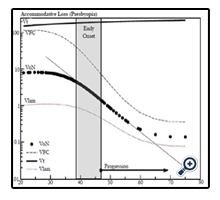Figure 7. The graphic shows the age related changes in lens’ fiber volume (μL) components. The total lens volume (Vt) , laminar layer volume, Vlam (indicated by the dotted line),VoN (solid closed circles), and VFC (dashed black line) are calculated as described in the text.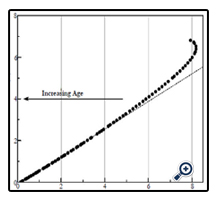Figure 8. Graphic portraying the ratio of ∂VoN (units μL) to ∂D (units diopters)(solid closed circles). The least-squares dashed line: 1.74 ± 0.17 μL/∂D indicates an age-dependent (left indicating arrow) loss.

Calculation of the Lens Fiber Cell - Laminar - Fluid Layer Volume (Vlam) and its Possible Effects on Loss of Accommodation

Use of the change in the lens CSA, as determined using our ellipsoid parameters, provides additional insights into the Hagen- Poiseuille-derived laminar fluid layer (Vlam). The calculated fiber cell laminar layer radial thickness is only 117 nm (below the wave length of visible light: 400 – 700 nm), compared to the 2.7 microns  of the fiber cell radius (Figure 5B), and would preclude microscopic detection.

In Figure 7, we compare the principle components of VoN: Vlam and VFC. The change in parameters with age is also shown in Figure 7. The results emphasize the changing relationship (age 40–50 years) of these important determined fluid volume parameters as an explanation for the dysfunctional optics (presbyopia). The VFC protein core is marked in blue in Figure 6 (and 9). VFC makes up the larger optical refractive component within the fiber cell, which presumably moves with the aid of both a fluid layer (Vlam) and compression forces (Pai). We can suppose that it is composed of fiber cell structural protein (e.g., cytosol crystallins, matrix protein, etc.). Beyond about age 45, the rate of VFC volume loss becomes nearly constant (0.055 μL/year), in alignment with the age when ∂D drops below +2D. Both VoN and Vlam may have lower protein levels. Figure 8 shows the ratio of VoN to ∂D with a least-squares slope value of 1.74 (SE ±0.03) μL/∂D (R² = 0.998).

Calculation of Possible Lens Drug Level Requirements to Restore Accommodation

The lens density includes about 31.5% protein . The major protein, alpha-crystallin,which comprises over 50% of the lens crystallins , with an average molar mass of 636kD, provides an estimate for the VoN volume mass equivalent (2.6 μg) of 4.09 pmoles of protein per 8.86 μL (e.g., at 21years). A reasonable endpoint for presbyopia treatment is the recovery +2∂D, which would require a minimal lens drug delivery of approximately 1.5p moles (3.4μL) to restore the optical fluid displacement volume that is responsible for accommodation.

Discussion

Fisher found that the lens resting in the dis-accommodated state has an anterior-to-posterior ratio (VaD/VpD shown in Figure 1) of approximately 0.41. This ratio is not random, but is required for efficient optical performance. Maximizing the anterior polar axis curvature needed for accommodation requires that a temporary fluid reservoir (VoN) within the lens be maintained outside the visual polar axis (i.e., the normal dis-accommodated state set for distance vision). Kortez and Handelman indirectly referenced our term VoN, with the observation that “…with internal movements during small accommodative changes. The net result of accommodation is a movement of lens mass in the anterior direction, in addition to change of curvature described above. Considering the nested layers of lens fibers and the compartmentalization of lens cytoplasm into these fibers, we assume that the net anterior lens movement results from redistribution of the fiber cytoplasm” . The fluid biomechanics described here are illustrated in Figure 9. The calculations do not consider the lens GRIN on accommodation . Figure 9 illustrates the fluid displacement as a conceptual overview. The displacement volume, VoN, is composed of fiber cell laminar protein (Vlam, gray color) and core protein (VFCore, blue color). Collectively, the ∂VaD and ∂VpD (labeled in Figure 9), which determine the total equatorial fluid reservoir, aid our understanding of presbyopia treatment. Aging factors affecting VoN are important for understanding the loss of accommodation. The linear slope shown in Figure 8 demonstrates an expected constant relationship of the calculated fluid displacement (VoN) with clinically determined accommodative amplitude (∂D), 1.74 ± 0.174 μL/∂D. The resting-state fluid reservoir resides largely within a fraction of the cortical proteins.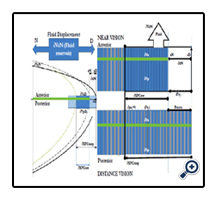Figure 9. Illustration of the underlying lens mechanism (D to N) of fluid displacement volume (VoN) associated with accommodation. The 21-year-old lens CSA (separately calculated D and N profiles) is shown on the left. Included with the lens profile are the directions (upper blue arrow) of the ∂VoN or fluid reservoir displacement, depending on D/N. The illustration denotes the cortical equatorial anterior and posterior regions (separated by the green bar). The lens profile includes simple representative bar diagrams (light/dark blue bars) placed at the equatorial region of the lens profile (at the D fluid reservoir location).The displacement volumes (D/N) are more detailed in the bar diagrams shown in the right figure,which are composed of fiber cell laminar (Vlam ) (gray) and core protein (Vlam and VFCore color designations, as detailed in Figure 6). The lower-right bardiagram – distance vision (D under zonular tension labelled Ue) –shows exposed gray bars(Vlam ). The upper-right bar diagram –near vision (N; no tension with Ue to KE conversion) –shows no exposed gray Vlam (displaced). The remaining ∂VoN is displaced to the polar axis of a certain refractive protein fraction of VFCore (∂VFCore ), aided by Vlam (see Discussion). The associated inward movementis ∂xN and the final equatorial axis is aN, as Pai approaches 0. The ∂XFCore is equal to the net inward movement of ∂xN , relative to ∂XFComp . Fiber cells beyond (or toward the inner lens nuclear region) are thought to be limited by zonular force displacement and are largely unaffected or inactive in terms of functional optics.

The accommodative amplitude (∂D) results from compression forces (Pai) applied to a limited (i.e., by external energy) fiber cell volume, VFComp, or the equatorial compression volume (gray plus blue colors). The changing fluid reservoir volume, equal to VoN, is contained within unique long lens fiber cells (that connect and direct the displacement from the equatorial to the polar visual region), analogous to Hagen-Poiseuille "pipes" or tubes . Increasing protein aggregation (η)  resists the flow, and coupled with a presumably fixed capsule inward pressure (Pai), negatively impacts the resulting magnitude of accommodation caused by a resulting reduced ∂xN (Figure 4). The results from these calculations indicate that the ∂xN/∂D remains nearly a constant value, 0.0213 ± 0.002 mm/∂D, or about a factor of two lower than the 0.05 mm/∂D reported in the literature [14, 45].

A finite level of lens KE (force times distance or work Nm) is available for achieving lens microfluidics displacement (< 1 erg at 44 years). The nucleus becomes highly stiff with age ; which makes any changes in nuclear volume difficult, if not impossible, with only a finite potential energy. Scheimpflug imaging has been used to determine the shape of the human lens nucleus during accommodation in five subjects. The researchers fitted a parametric model of the cross-sectional geometry to the gradient of the Scheimpflug images, using the Hough transform. The volume of the nucleus showed no significant change during accommodation in any of the subjects . Finite element method studies, which are strictly structural analysis [16, 21, 35, 47] with no consideration for lens anatomy (fiber cell pipes or fluidics), have examined the force distribution applied to the lens for accommodation. The results would imply a more significant role of the lens cortical volume change immediately adjacent to the equatorial zonular attachment points. Operational optical function via fluidics, as developed here, would probably exclude the larger inner nucleus mass from a significant role in accommodation. The outermost cortical proteins are relatively newly synthesized (i.e., less stiff) when compared with older, centrally located nuclear proteins [17, 48, 49]. The comparatively stiffer inner nucleus  does provide a necessary flow boundary (opposing force) for the more efficient fluid movement noted in the literature (i.e., lower energy requirements with less lens mass). The Hagen-Poiseuille term, Pai, is proportional to inward force and 1/η (i.e., lens viscosity) [21, 51-53]. Near vision (i.e., increased polar axis curvature) is ultimately based on the balance between outward lens pressure (Pao) and inward capsule pressure (Pai). Counterintuitively, our lens distance vision is in a resting, higher-energy state (i.e., under zonular tension), whereas our near vision is energetically passive (i.e., uses stored energy). The zonular tension (ergs) on the capsule and underlying fiber cells creates a potential energy (Ue, marked in Figure 9). The capsule pressure molds the gel-like material properties of the lens, but the lens fiber cells adds a “spring-like” inward force that is necessary for restoring near vision . Importantly (for near vision), the conversion of Ue into kinetic energy (KE), as marked in Figure 9, provides the necessary “work” for accommodation when the ciliary muscle contraction releases the zonular tension. The differential force of compression displaces a mass within an equivalent volume to VoN . With energy release (i.e., over the radial distance ∂xN, see Figure 9), mass within volume VoN is displaced into the optical path affecting lens curvature . The intrinsic laminar layer volume (Vlam) is an important conceptual part of the lens fluidics study described by the Hagen- Poiseuille equation (EQN 35) for laminar (non-turbulent) flow. We used laminar layer calculations to determine the volume extent of VFComp and VFC. With age, the decreasing laminar layer can impede required levels of microfluidic VoN movement to achieve sufficient ∂D for accommodation. Knowledge of changes in the lens cross-sectional areas for fluid displacement provides the calculation of the laminar layer (labeled Vlam in Figures 5A, 8A, and 9) concept within the fiber cell. This same numerical method is used to account for measured high-viscosity blood flow (i.e., the Fahraeus-Lindqvist Effect) . Blood Red cell movement through the micro-vascular network is explained by localizing serum fluid at the capillary wall. Vlam, by analogy, occurs adjacent to the fiber cell membrane, facilitating ∂VFCore (a part of VoN) movement (∂xN) toward the central visual axis. Importantly, Vlam may act to “lubricate” movement of the fiber cell protein “core” volume (VFCore). The lens fiber cell diameter is remarkably similar to the Fahraeus- Lindqvist-calculated optimal diameter of 10μm, and it may thereby implicate a defined role in the lens structural function. The process of using lubrication would lower the energy requirements for accommodation. As zonular tension is released, the core protein, acting as an optical element (with a higher refractive index than aqueous) displaces the fiber cells low-viscosity laminar layer (i.e., lower index) with an extra cellular volume exchange (e.g., at 21years, Vlam < 1.6 μL per lens), providing a mechanical fluid (e.g., AQP0 ) facilitated diffusion. Candia and Alvarez (2008) “… propose the possible existence of a fluid flow between the lens and the intraocular fluids during accommodation. The resulting fiber cell exchange, providing nutrient influx (e.g., drugs) and efflux-removing waste products (e.g., lactate) may be required to maintain lens transparency s. The restricted nutrient exchange provided by Vlam (< 0.14 μL at age 55years) could also negatively impact lens clarity (i.e., cataract formation). Lens compression, with available forces, is also illustrated in Figure 9 (left blue bar) in the larger lens profile [59, 60]. As Figure 9 (i.e., the calculated 21-year-old lens profile) illustrates, the lens VFComp region resides within the more familiar anatomical outer lens cortical region. For working lens biomechanics, the compression volumes (VFC) are equal at the equatorial and polar axes; in effect, they are almost double the calculated VFComp volume shown in Figure 9. The action delivers an equivalent VoN to the central optical region (illustrated in Figure 9). The calculated interrelationship to ∂xN and ∂XFCore is also illustrated in Figure 9. This is the net distance over which lens compression occurs (∂XFComp), as shown in the CSA lens profile. Note that the internal equatorial VFCore may functionally maintain the structural integrity of the fiber cell, where it may prevent the fiber cells from collapsing due to applied inward compression forces (Pai). The increasing lens modulus progressivelyaffects inward movement (i.e., xN-fitted values, see Figure 4). The actual ∂xN (with age) to achieve this geometry is restricted by increasing the internal lens modulus or stiffness; however, our calculation methods do not use empirically derived modulus values directly , but can be inferred. When the inward force-motion(KE) approaches zero, ∂XFComp (i.e., the calculated values, see Figure 7) inward motion ceases, as illustrated in Figure 9 (upper bar compression), with a final limiting ∂D being achieved. These calculations, when taken together, correlate the effects of fluid movement on the lens geometry (i.e., structure) and optics (i.e., function). The evolved lens adaptive optics system depends on adequate and rapid fluid displacement to achieve neuro-responsive dynamic optical changes. For near vision, the lens cells must allow fluid displacement from the equatorial vertex to the central optical path.

A presbyopia treatment requires some knowledge of the extent of the optical defect within the lens. The question to be addressed is whether the whole lens is dysfunctional or only part of it, in order to determine “… how potent a topical drug might be needed to treat presbyopia.” By age 45, the total lens protein mass is approximately 70mg. Assuming a 635 mass/kDa (i.e., alpha-crystallin) [61, 62], the equivalent drug delivery for a presbyopia treatment would be about 95 pmoles/lens just for repair of (nuclear) disulfide linked protein (e.g., PSSP). This amount does not consider metabolite oxidation (e.g., glutathione disulfides or GSSG) . A value of 95 pmoles/lens to the lens would likely preclude a topical pharmaceutical treatment for presbyopia within a short time span. The primary application of these methods may provide us with some insights as to whether the cortical region is a viable target for directing presbyopia treatments, and, if so, what level of drug delivery (i.e., in terms of effects on duration of treatment) might be needed to effect such a presbyopia treatment.

Encore Vision completes a Phase I/II Trial for Presbyopia in the USA (NCT02516306)

A Phase 1/2 Prospective, Randomized, Double-Masked, Placebo-Controlled, Multicenter Study was performed to Evaluate the Safety and Efficacy of EV06 Ophthalmic Solution (Lipoic Acid Choline Ester, 1.5%), in Improving Distance Corrected Near Vision in Subjects With Presbyopia. The trial included 75 (N=25 Placebo) patients that received BID. EV06 provided a visual acuity improvement of approximately 2-Snellen lines and an associated increase of lens refractive power . The distance corrected near visual acuity (DCNVA) results showed a significant effect [P<0.0001]). Only small amounts of the active agent EV06 are delivered (21 pmoles) over the 91-day treatment period (separate ADME-PK study). The positive NCT02516306 (clinicaltrials.gov) outcome appears to confirm that the cortical lens volume, and not the nucleus, is the most likely lens target for the active agent in the treatment of presbyopia.

Conclusions

• Ellipsoidal major and minor axis parameters for near vision were derived from the best fit to the clinical optical measurements to account for increased lens modulus.
• Accommodation requires changes in lens geometry (determined through oblate ellipsoid parameters), which are affected by relatively minor but constant internal fluid displacement in cortical lens fiber cells(termed VoN) of 1.74 ± 0.17 μL/D. The inward equatorial distance change per diopter remains at a near-constant value of 21.2 ± 0.002 microns/∂D, despite the measured increases in the lens Young’s modulus (Pa).
• Targeting drugs for presbyopia treatment to the small intracellular laminar lens volume element may require delivery of as little as 1.9 pmoles to an active lens.

Acknowledgments

Encore Vision, Inc. provided research support. Portions of this study were presented in abstract form at the Association for Research in Vision and Ophthalmology, Annual Meeting, Orlando, FL, on May 3, 2014.

References

1. Taylor VL, Al-Ghoul KJ, Lane CW, Davis VA, Kuszak JR, et al., (1996) Morphology of the normal human lens. Invest Opthalmol Vis Sci. 37(7): 1396-1410.
2. Kuszak JR, Mazurkiewicz M, Zoltoski R (2006) Computer modeling of secondary fiber development and growth: I. Nonprimate lenses. Mol Vis. 12: 251–270.
3. Kuszak JR, Zoltoski RK (2005) The mechanism of accommodation at the lens fiber level Focus on Eye Research. Nova Science Publishers, Inc, New York.
4. Werner L, Trindade F, Pereira F, Werner L (2000) Physiology of accommodation and presbyopia. Arq Bras Oftalmol. 63(6): 487–493.
5. Beers AP, van der Heijde GL (1996) Age-related changes in the accommodation mechanism. Optom Vis Sci. 73(4): 235–242.
6. Glasser A, Croft MA, Kaufman PL (2001) Aging of the Human Crystalline Lens and Presbyopia. Int Ophthalmol Clin. 41(2): 1–15.
7. Helmholtz H (1855) Ueber die Accommodation des Auges. Graefes Arch Clin Exp Ophthalmol, Springer-Verlag . 2(2): 1–74.
8. Mohamed A, Sangwan V, Augusteyn R (2012) Growth of the human lens in the Indian adult population: Preliminary observations. Indian J Ophthalmol. 60(6): 511–512.
9. Fisher RF (1973) Presbyopia and the changes with age in the human crystalline lens. J Physiol. 228(3): 765–779.
10. Miesbauer LR, Zhou X, Yang Z, Sun Y, Smith DL, et al., (1994) Post-translational modifications of water-soluble human lens crystallins from young adults. J Bio Chem. 269(17): 12494–12502.
11. Cumming RC, Andon NL, Haynes PA, Park M, Fischer WH, et al., (2004) Protein disulfide bond formation in the cytoplasm during oxidative stress. J Bio Chem. 279(21): 21749–21758.
12. Spector A, Roy D (1978) Disulfide-linked high molecular weight protein associated with human cataract. Proc Natl Acad Sci U S A. 75(7): 244–3248.
13. Harding JJ (1973) Disulpbide Cross-linked Protein of High Molecular Weight in Human Cataractous Lens. Exp Eye Res. 17(4): 377–383.
14. Dubbelman M, van der Heijde GL, Weeber HA, Vrensen GF (2003) Changes in the internal structure of the human crystalline lens with age and accommodation. Vision Res. 43(22): 2363–2375.
15. Kasthurirangan S, Markwell EL, Atchison DA, Pope JM (2008) In vivo study of changes in refractive index distribution in the human crystalline lens with age and accommodation. Invest Ophthalmol Vis Sci. 49(6): 2531–2540.
16. Weeber HA, van der Heijde RGL (2007) On the relationship between lens stiffness and accommodative amplitude. Exp Eye Res. 85(5): 602–607.
17. Heys KR, Cram SL, Truscott, RJ (2004) Massive increase in the stiffness of the human lens nucleus with age: the basis for presbyopia? Mol Vis. 10: 956–963.
18. Kuszak JR, Zoltoski RK, Tiedemann CE (2004) Development of lens sutures. Int J Dev Biol. 48(8-9): 889–902.
19. Koretz JF, Handelman GH (1982) Model of the accommodative mechanism in the human eye. Vision Res. 22(8): 917–927.
20. Van de Sompel D, Kunkel GJ, Hersh PS, Smits AJ (2010) Model of accommodation: Contributions of lens geometry and mechanical properties to the development of presbyopia. J Cartaract Refract Surg. 36(11): 1960–1971.
21. Burd HJ, Judge SJ, Cross JA (2002) Numerical modelling of the accommodating lens. Vis Res. 42(18): 2235–2251.
22. Reilly MA, Ravi N (2010) A geometric model of ocular accommodation. Vision Res. 50(3): 330–336.
23. Kasprzak HT (2000) New approximation for the whole profile of the human crystalline lens. Ophthalmic Physiol Opt. 20(1): 31–43.
24. Anderson HA, Hentz G, Glasser A, Glasser AA, Stuebing KK, et al., (2008) Minus-lens-stimulated accommodative amplitude decreases sigmoidally with age: a study of objectively measured accommodative amplitudes from age 3. Invest Ophthalmol Vis Sci. 49(7): 2919–2926.
25. Ravi N, Wan KT, Swindle K, Hamilton PD, Duan G (2006) Development of techniques to compare mechanical properties of reversible hydrogels with spherical, square columnar and ocular lens geometry. Polymer. 47(11): 4203–4209.
26. Lin YL, Wang DM, Lu WM, Lin YS, Tung KL (2008) Compression and deformation of soft spherical particles. Chem Eng Sci. 63: 195–203.
27. Farnsworth PN, Shyne SE (1979) Anterior zonular shifts with age. Exp Eye Res. 28(3): 291–297.
28. Manns F, Parel JM, Denham D, Billotte C, Ziebarth N, et al., (2007) Optomechanical Response of Human and Monkey Lenses in a Lens Stretcher. Invest Ophthalmol Vis Sci. 48: 3260–3269.
29. Marussich L, Manns F, Nankivil D, Maceo Heilman B , Yao Y, et al., (2015) Measurement of Crystalline Lens Volume During Accommodation in a Lens Stretcher. Invest Ophthalmol Vis Sci. 56(8): 4239–4248.
30. Burd HJ, Wilde GS, Judge SJ (2006) Can reliable values of Young’s modulus be deduced from Fisher’s (1971) spinning lens measurements? Vision Res. 46(8-9): 1346–1360.
31. Dubbelman M, van der Heijde GL, Weeber HA (2005) Change in shape of the aging human crystalline lens with accommodation. Vision Res. 45(1): 117–132.
32. Rosales P, Dubbelman M, Marcos S, van der Heijde R (2006) Crystalline lens radii of curvature from Purkinje and Scheimpflug imaging. J Vision. 6(10): 1057–1067.
33. Fisher RF (1969) Elastic constants of the human lens capsule. J Physiol. 201(1): 1–19.
34. Hermans EA, Pouwels PJW, Kuijer JPA, van der Heijde RGL, Heethar RM, et al., (2008) Constant Volume of the Human Lens and Decrease in Surface Area of the Capsular Bag during Accommodation: An MRI and Scheimpflug Study. Invest Ophthalmol Vis Sci. 50(1): 281–289.
35. Belaidi A, Pierscionek BK (2007) Modeling internal stress distributions in the human lens: can opponent theories coexist? J Vis. 7(11): 1–12.
36. Teichman J, Holzer J, Balko B, Fisher B, Buckley L (2013) Gradient Index Optics at DARPA. IDA, Alexandria: virginia.
37. Navarro R, Palos F, González LM (2007) Adaptive model of the gradient index of the human lens. II. Optics of the accommodating aging lens. J Opt Soc Am A Opt Image Sci Vis. 24(9): 2911–2920.
38. Pierscionek BK (1990) Presbyopia–effect of refractive index. Clin Exp Optometry. 73(1): 23–30.
39. Garner LF, Yap MK (1997) Changes in ocular dimensions and refraction with accommodation. Ophthalmic Physiol Opt. 17(1): 12–17.
40. Bron AJ, Vrensen G, Koretz J, Maraini G, Harding JJ (2000) The ageing lens. Ophthalmologica. 214(1): 86–104.
41. Gullstrand A (1911) How I found the mechanism of intracapsular accommodation. Nobel Lectures.
42. Peschek J, Braun N, Franzmann TM, Georgalis Y, Haslbeck M, et al., (2009) The eye lens chaperone alpha-crystallin forms defined globular assemblies. Proc Natl Acad Sci USA. 106(32): 13272–13277.
43. Tiffany JM, Koretz JF (2002) Viscosity of alpha-crystallin solutions. Int J Biol Macromol. 30(3-4): 179–185.
44. Jones CEC, Atchison DAD, Pope JMJ (2007) Changes in lens dimensions and refractive index with age and accommodation. Optom Vis Sci. 84(10): 990–995.
45. Hermans E, Dubbelman M, van der Heijde R, Heethaar R (2007) The shape of the human lens nucleus with accommodation. J Vision. 7(10):16.1–16.10
46. Weeber HA, van der Heijde RGL (2008) Internal deformation of the human crystalline lens during accommodation. Acta Ophthalmol. 86(6): 642–647.
47. Weeber HA, Eckert G, Pechhold W, van der Heijde RG (2007) Stiffnessgradient in the crystalline lens. Graefes Arch Clin Exp Ophthalmol. 245(9): 1357–1366.
48. Hozic A, Rico F, Colom A, Buzhynskyy N, Scheuring S (2012) Nanomechanical characterization of the stiffness of eye lens cells: a pilot study. Invest Ophthalmol Vis Sci. 53(4): 2151–2156.
49. Judge SJ, Burd HJ (2002) Modelling the mechanics of accommodation and presbyopia. Ophthalmic Physiol Opt. 22(5): 397–400.
50. Bocskai Z, Bojtár I (2013) Biomechanical modelling of the accommodation problem of human eye. Per Pol Civil Eng. 57(1): 3–7.
51. Weeber HA, Eckert G, Soergel F, Meyer CH, Pechhold W, et al., (2005) Dynamic mechanical properties of human lenses. Exp Eye Res. 80(3): 425–434.
52. Olesen LH, Okkels F, Bruus H (2004) Topology optimization of Navier- Stokes flow in microfluidics. ECCOMAS. Jyvaskyla.
53. Makinde OD (2005) Collapsible tube flow: a mathematical model. Rom J Phys. 50(5/6): 493–506.
54. Sharan M, Popel AS (2001) A two-phase model for flow of blood in narrow tubes with increased effective viscosity near the wall. Biorheology. 38(5-6): 415–428.
55. Gerometta R, Zamudio AC, Escobar DP, Candia OA (2007) Volume change of the ocular lens during accommodation. Am J Physiol Cell Physiology. 293(2): C797–C804.
56. Candia OA, Alvarez LJ (2008) Fluid transport phenomena in ocular epithelia. Prog Retin Eye Res. 27(2): 197–212.
57. Donaldson P, Kistler J, Mathias RT (2001) Molecular solutions to mammalian lens transparency. News Physiol Sci. 16: 118–123.
58. Katz AI, Chen Y, Moreno AH (1969) Flow through a collapsible tube: experimental analysis and mathematical model. Biophys J. 9(10): 1261–1279.
59. Grotberg JB, Jensen OE (2004) Fluid flow in collapsible elastic tubes: A three-dimensional numerical model. Annu Rev Fluid Mech. 36: 121–147.
60. Thomson JA, Augusteyn RC (1983) αm-Crystallin: the native form of the protein? Exp Eye Res. 37(4): 367–377.
61. Siezen RJ, Berger H (1978) The quaternary structure of bovine alpha-crystallin. Size and shape studies by sedimentation, small-angle X-ray scattering and quasi-elastic light scattering. Eur J Biochem. 91(2): 397–405.
62. Harding JJ (1970) Free and protein-bound glutathione in normal and cataractous human lenses. Biochem J. 117(5): 957–960.
63. Katada Y, Negishi K, Watanabe K, Shigeno Y, Saiki M, et al., (2016) Functional Visual Acuity of Early Presbyopia. PLoS ONE. 11(3): e0151094.
64. Garner WH, Garner M, Crawford KS, Burns W (2014) Dioptin™ Eye Drop to Treat Presbyopia: corneal penetration and ocular pharmacokinetics. Invest Ophthalmol Visual Sci. 55: 3766–3766.
65. Langner S, Martin H, Terwee T, Kruger PC, Hosten N, et al., (2010) 7.1 T MRI to Assess the Anterior Segment of the Eye. Invest Ophthalmol Vis Sci. 51(12): 6575–6581.

### Site Map

#### Total Visitors### Get in Touch

SciDoc Publishers
16192 Coastal Highway
Lewes, Delaware 19958
Tel :+1-(302)-703-1005
Fax :+1-(302)-351-7355
Email: contact.scidoc@scidoc.org